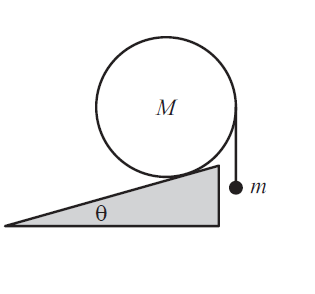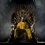# Exercise 2.31 cylinder and hanging massA uniform cylinder of mass $M$ sits on a fixed plane inclined at an angle $\theta$. A string is tied to the cylinder’s rightmost point, and a mass $m$ hangs from the string, as shown in Figure above. Assume that the coefficient of friction between the cylinder and the plane is sufficiently large to prevent slipping.

What is $m$, in terms of $M$ and $\theta$, if the setup is static?Note by Beakal Tiliksew
7 years, 1 month ago

This discussion board is a place to discuss our Daily Challenges and the math and science related to those challenges. Explanations are more than just a solution — they should explain the steps and thinking strategies that you used to obtain the solution. Comments should further the discussion of math and science.

When posting on Brilliant:

• Use the emojis to react to an explanation, whether you're congratulating a job well done , or just really confused .
• Ask specific questions about the challenge or the steps in somebody's explanation. Well-posed questions can add a lot to the discussion, but posting "I don't understand!" doesn't help anyone.
• Try to contribute something new to the discussion, whether it is an extension, generalization or other idea related to the challenge.

MarkdownAppears as
*italics* or _italics_ italics
**bold** or __bold__ bold
- bulleted- list
• bulleted
• list
1. numbered2. list
1. numbered
2. list
Note: you must add a full line of space before and after lists for them to show up correctly
paragraph 1paragraph 2

paragraph 1

paragraph 2

[example link](https://brilliant.org)example link
> This is a quote
This is a quote
    # I indented these lines
# 4 spaces, and now they show
# up as a code block.

print "hello world"
# I indented these lines
# 4 spaces, and now they show
# up as a code block.

print "hello world"
MathAppears as
Remember to wrap math in $$ ... $$ or $ ... $ to ensure proper formatting.
2 \times 3 $2 \times 3$
2^{34} $2^{34}$
a_{i-1} $a_{i-1}$
\frac{2}{3} $\frac{2}{3}$
\sqrt{2} $\sqrt{2}$
\sum_{i=1}^3 $\sum_{i=1}^3$
\sin \theta $\sin \theta$
\boxed{123} $\boxed{123}$

Sort by:alt text

In the above image, I have marked out the forces on the cylinder, and also, I have removed the mass $\displaystyle m$.

Firstly, if the system is static, then the tension in the string must be $\displaystyle mg$, so that there is equilibrium for the mass $\displaystyle m$.

For attaining rotational equilibrium,
Considering the torque about the contact point of the cylinder and the inclined plane,

$Mg(R\sin\theta) = T(R-R\sin\theta)$

The torque due to the Normal Reaction and the Frictional force, is zero, since those forces pass through the contact point (the point which we are considering the torque about)

$\Rightarrow Mg(R\sin\theta) = mgR(1-\sin\theta)$

$\Rightarrow M\sin\theta = m(1-\sin\theta)$

$\Rightarrow \boxed{m = \frac{M\sin\theta}{1-\sin\theta}}$

- 7 years, 1 month ago

Anish, which software do you use to draw diagrams?

- 7 years, 1 month ago

I use Photoshop CS5

- 7 years, 1 month ago

Oh, I don't have it. Could you recommend something else with which I can put a decent diagram?

- 7 years, 1 month ago

Paint is not bad, if you just draw simple diagrams.

- 7 years, 1 month ago

Thanks, Anish! : )

- 7 years, 1 month ago

nice explanation

- 7 years ago